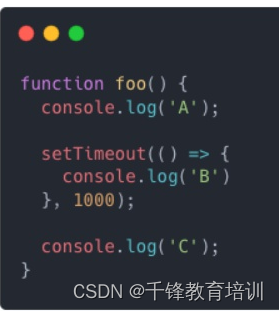# 前端面试题：JavaScript常见面试题

01、console.log(1 < 2 < 3) 返回什么？

02、如何将两个数组合并在一起？

array.concat()

``````const array1 = ['a', 'b', 'c'];
const array2 = ['d', 'e', 'f'];
const array3 = array1.concat(array2);
console.log(array3);  // expected output: Array ["a", "b", "c", "d", "e", "f"]
``````

``````let array1 = [1,2];
let array2 = [3,4,5];
let mergedArray = [...array1, ...array2];
console.log(mergedArray) // [1,2,3,4,5];
``````

03、array.map和array.forEach有什么区别？

``````const a = [1, 2, 3];
const doubled = a.forEach((num, index) => {
// Do something with num and/or index.
});
console.log(doubled) // undefined
const a = [1, 2, 3];
const doubled = a.map((num) => {
return num * 2;
});
// doubled = [2, 4, 6]
``````

04、控制台的打印结果是什么？05、var、let 和 const 有什么区别？

const 是用于声明常量的关键字，它基本上与 let 关键字非常相似，不同之处在于，它创建对值的只读引用并且不能重新分配值。例如：

``````const a = 10;
a = 20;  // Type error as value cannot be reassigned
``````